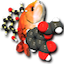"babel ... -o svg" - Two "svg" Tag Levels

Q

Why am getting two "svg" tag levels in SVG source code generated by the "babel" command?

✍: FYIcenter.com

AIf you are using Open Babel 2.4.1 or higher, you will notice that there are two "svg" tag levels in SVG source code generated by the "babel" command.

The main reason for using two "svg" tag levels for including multiple molecules in a single SVG file so that:

• The outer level "svg" tag defines the larger viewing area that is divided into rows and columns to host individual molecules.
• The inner level "svg" tag defines a smaller viewing area to host single molecule.

For example, the following command generate a SVG picture contains 66 molecules:

```fyicenter\$ babel mol-*.sdf multi-molecules.svg

66 molecules converted
1388 audit log messages
```

If you open the SVG file, multi-molecules.svg, in a text editor, you will see the following two-level "svg" tag structure:

```<svg version="1.1" id="topsvg"
xmlns:cml="http://www.xml-cml.org/schema" x="0" y="0" width="100%" height="100%" viewBox="0 0 100 88">
<title>Multiple Molecules - Open Babel Depiction</title>
<rect x="0" y="0" width="100" height="88" fill="white"/>

<g transform="translate(0,0)">
<svg width="11.1111" height="11.1111" x="0" y="0" viewBox="0 0 425.571 500.005"
font-family="sans-serif" stroke="rgb(0,0,0)" stroke-width="2"  stroke-linecap="round">
<line x1="247.8" y1="160.0" x2="282.5" y2="180.0" stroke="rgb(0,0,0)"  stroke-width="2.0"/>
<line x1="178.6" y1="160.0" x2="155.2" y2="173.5" stroke="rgb(0,0,0)"  stroke-width="2.0"/>
...
</svg>
</g>

<g transform="translate(11.111111,0.000000)">
<svg width="11.111111" height="11.111111" x="0" y="0" viewBox="0 0 426.667619 477.075053"
font-family="sans-serif" stroke="rgb(0,0,0)" stroke-width="2.000000"  stroke-linecap="round">
<line x1="248.0" y1="100.0" x2="282.7" y2="80.0" stroke="rgb(0,0,0)"  stroke-width="2.0"/>
<line x1="282.7" y1="80.0" x2="306.1" y2="93.5" stroke="rgb(0,0,0)"  stroke-width="2.0"/>
...
</svg>
</g>

</svg>
```

As you can see, the two-level "svg" tag structure works nicely for multiple molecules:

• The outer "svg" tag with an id of "topsvg" controls the entire picture with a viewBox of "0 0 100 88".
• The first inner "svg" tag holds the first molecule occupying an area defined as (width="11.1111" height="11.1111") with no transformation, "translate(0,0)". So it stays near the (0,0) corner, top left corner.
• The first inner "svg" tag holds the second molecule occupying an area defined as (width="11.1111" height="11.1111"), same size as the first molecule. But it has a transformation of "translate(11.111111,0.000000)". So it is moved horizontally to the second column of the first row.
• With each molecule defined as (width="11.1111" height="11.1111"), the entire viewBox of "0 0 100 88" will hold 9 molecules horizontally and 8 molecules vertically. In other words, all 66 molecules will be displayed in 8 rows and 9 columns.

However, for a single molecule, Open Babel still uses this two-level "svg" structure, which is unnecessary and confusing.

Also note that if you have a script that parses SVG files generated from older versions of Open Babel, your script may fail. Because Open Babel 2.3.1 was generating a one-level "svg" structure if there is only one molecule.

2020-07-22, 624👍, 0💬